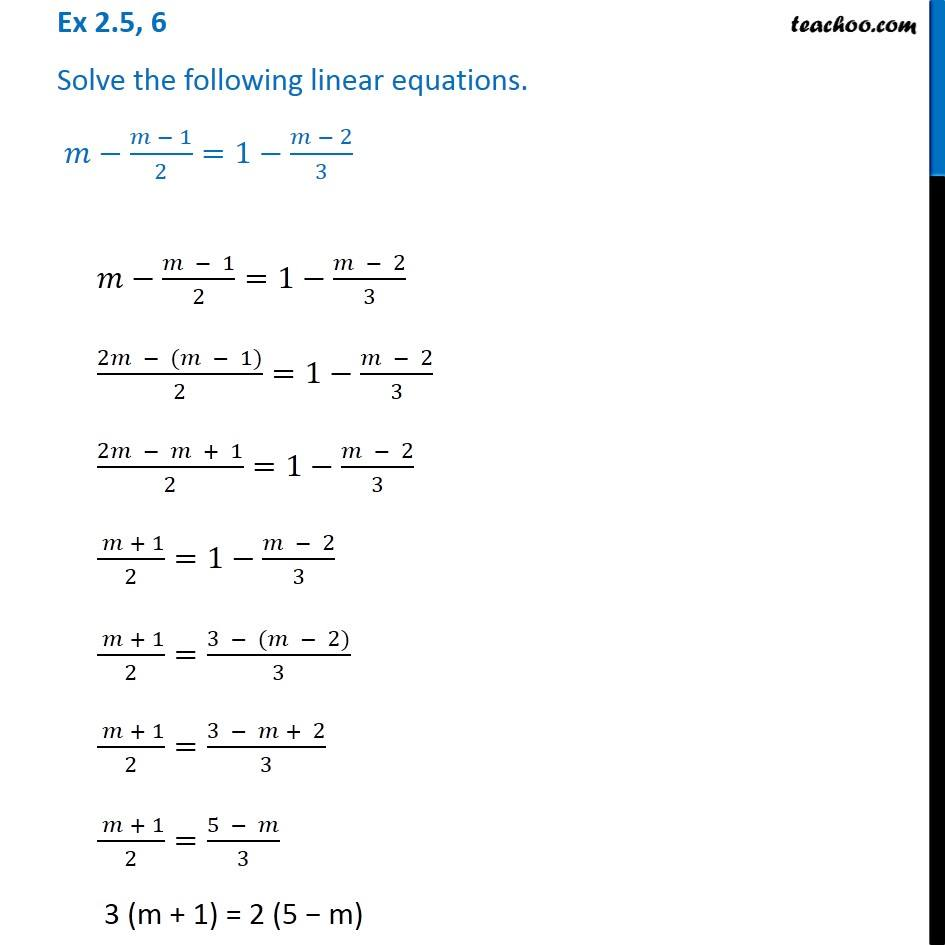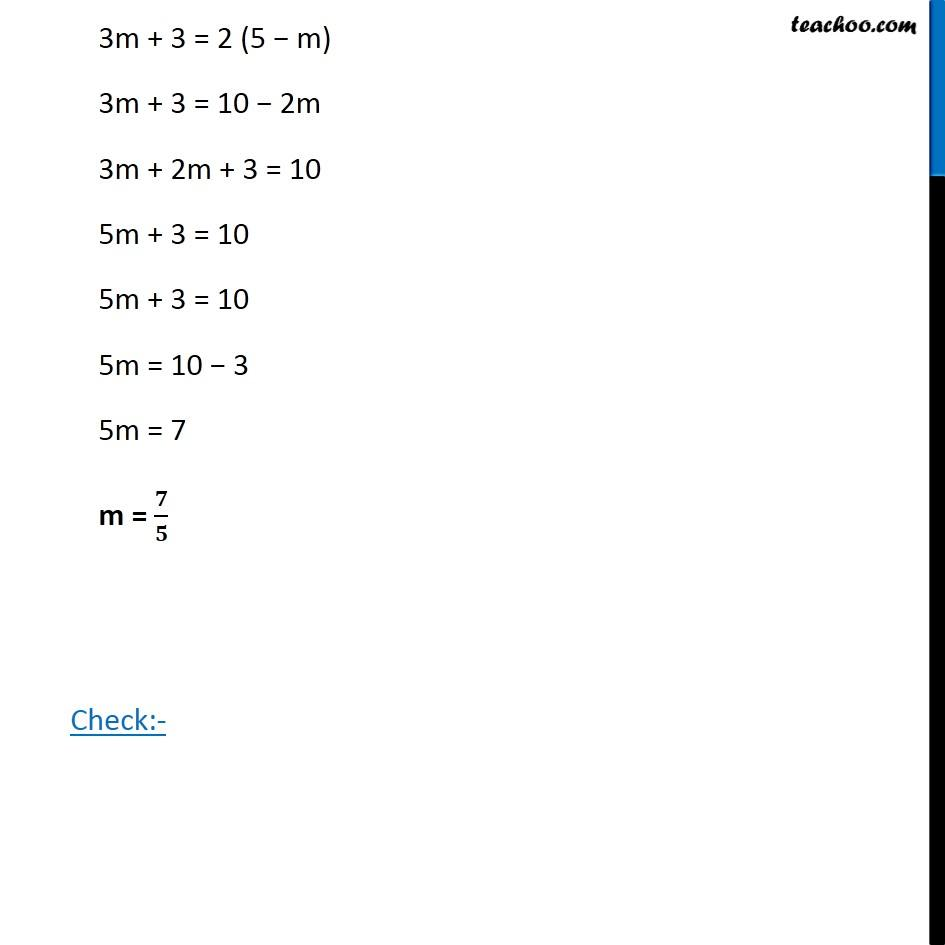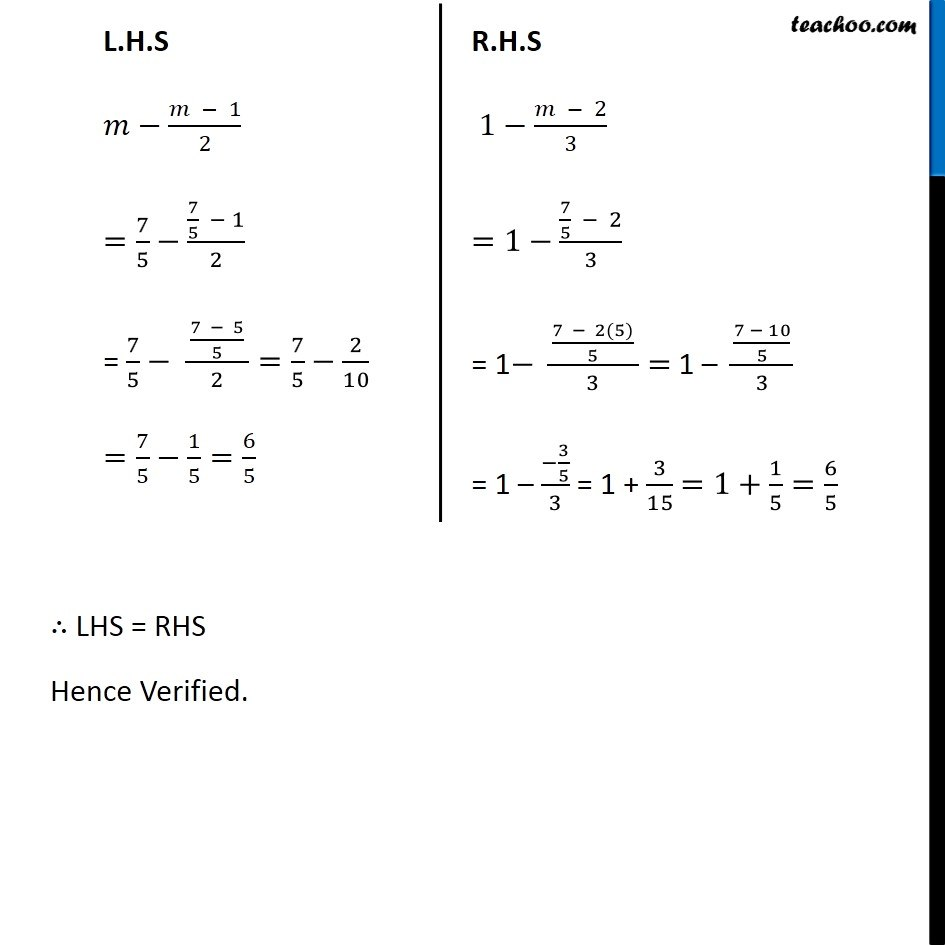Ex 2.5

Chapter 2 Class 8 Linear Equations in One Variable
Serial order wiseGet live Maths 1-on-1 Classs - Class 6 to 12

### Transcript

Ex 2.5, 6 Solve the following linear equations. 𝑚−(𝑚 − 1)/2=1−(𝑚 − 2)/33m + 3 = 2 (5 − m) 3m + 3 = 10 − 2m 3m + 2m + 3 = 10 5m + 3 = 10 5m + 3 = 10 5m = 10 − 3 5m = 7 m = 𝟕/𝟓 Check:- L.H.S 𝑚−(𝑚 − 1)/2 =7/5−(7/5 − 1)/2 = 7/5− ( (7 − 5)/5 )/2=7/5−2/10 =7/5−1/5=6/5 R.H.S 1−(𝑚 − 2)/3 =1−(7/5 − 2)/3 = 1− ( (7 − 2(5))/5 )/3= 1 – ((7 − 10)/5)/3 = 1 – (−3/5)/3 = 1 + 3/15 =1+1/5=6/5 ∴ LHS = RHS Hence Verified.